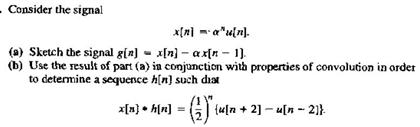# Question Consider the signal x[n] =alpha nu[n] Sketch the signal g[n] = x[n] - alpha x[n - 1] Use the result of part (a)in conjunction with properties of convolution in order to determine a sequence h[n] such x[n] + h[n] =(1/2)n{u[n + 2] -u[n - 2]}.36C9RE The Asker · Electrical EngineeringTranscribed Image Text: Consider the signal x[n] =alpha nu[n] Sketch the signal g[n] = x[n] - alpha x[n - 1] Use the result of part (a)in conjunction with properties of convolution in order to determine a sequence h[n] such x[n] + h[n] =(1/2)n{u[n + 2] -u[n - 2]}.
More
Transcribed Image Text: Consider the signal x[n] =alpha nu[n] Sketch the signal g[n] = x[n] - alpha x[n - 1] Use the result of part (a)in conjunction with properties of convolution in order to determine a sequence h[n] such x[n] + h[n] =(1/2)n{u[n + 2] -u[n - 2]}.